﻿ 基于模糊控制三电平Boost直流变换器输出电容均压方法 Output Capacitor Voltage Balance Control Method of Three-Level Boost DC-DC Converter Using Fuzzy Controller

Open Journal of Circuits and Systems
Vol. 08  No. 03 ( 2019 ), Article ID: 32161 , 11 pages
10.12677/OJCS.2019.8383008

Output Capacitor Voltage Balance Control Method of Three-Level Boost DC-DC Converter Using Fuzzy Controller

Lu Huang1, Yu Fang2, Songyin Cao2

1Yangzhou Haitong Electronic Technology Co. Ltd., Yangzhou Jiangsu

2College of Information Engineering, Yangzhou University, Yangzhou Jiangsu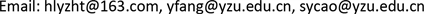Received: Aug. 27th, 2019; accepted: Sep. 9th, 2019; published: Sep. 16th, 2019ABSTRACT

Aiming at the neutral-point (NP) imbalance and the influence of the modulation strategy of the post-stage inverters on the neutral-point potential when the three-level Boost DC converter is used in the three-phase non-isolated grid-connected PV power generation inverters to start the voltage-building process, a fuzzy control strategy is proposed to realize the neutral-point potential balance at the power level of the three-level Boost DC converter. The duty cycle ratio of the switch of three-level Boost DC converter can be adaptively changed by using the fuzzy controller. Not only in the start-up process (when the DC bus is voltage-building), but also in the stable operation of grid-connected inverters, the voltages across output capacitors can be equalized and good dynamic and static performance is obtained. In this paper, a fuzzy controller is designed for three-level Boost DC converter, and the control rules are given. Finally, the feasibility and reliability of the method are verified by simulation and experiment.

Keywords:Three-Level Boost Converter, Neutral-Point Potential Balance, Fuzzy Control

1扬州海通电子科技有限公司，江苏 扬州

2扬州大学信息工程学院，江苏 扬州1. 引言

2. 中点电位不平衡原因分析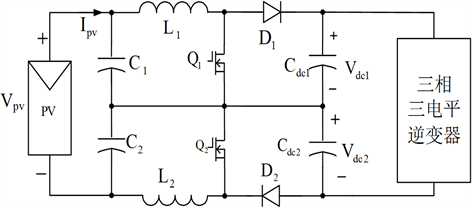Figure 1. Three-phase non-isolated photovoltaic grid-connected inverter system

3. 模糊控制器的原理及设计

3.1. 模糊控制器的原理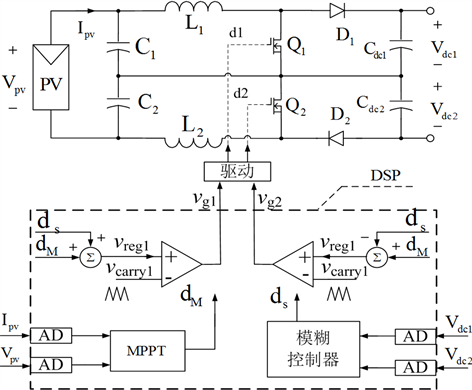Figure 2. The system schematic diagram based on fuzzy controller

d1与d2的形成如图2所示，模糊控制器输出dS，MPPT控制器输出dM。将dM和dS分别相加减得到调制波信号vreg1和vreg2，vreg1和vreg2分别与载波信号vcarry1和vcarry2进行比较(vcarry1和vcarry2具有相同的周期和相位)，得到PWM脉冲控制信号vg1和vg2，再经驱动电路控制开关管Q1和Q2的导通与关断。基于这种模糊控制器的控制系统能在跟踪光伏组件最大功率点的同时，实现直流输出两电容的均压控制。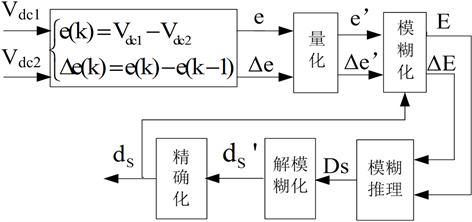Figure 3. The logic structure of fuzzy controller

3.2. 精确量的确定及量化

1) e区间的确定和量化

2) ∆e区间的确定和量化

3) dS区间的确定和量化

3.3. 偏差、偏差变化率和输出量的模糊化

1) 偏差e的模糊化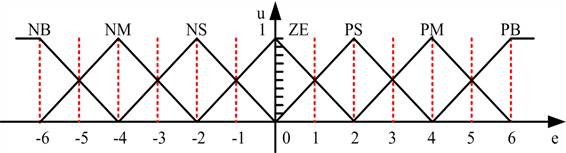Figure 4. The membership function of e

2) 偏差变化率∆e的模糊化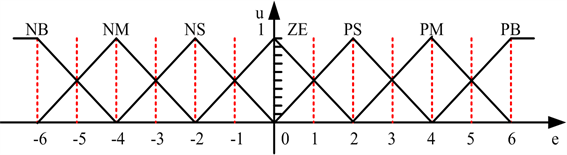Figure 5. The membership function of ∆e

3) 输出量dS的模糊化

3.4. 模糊控制规则的确定

4. 仿真和实验结果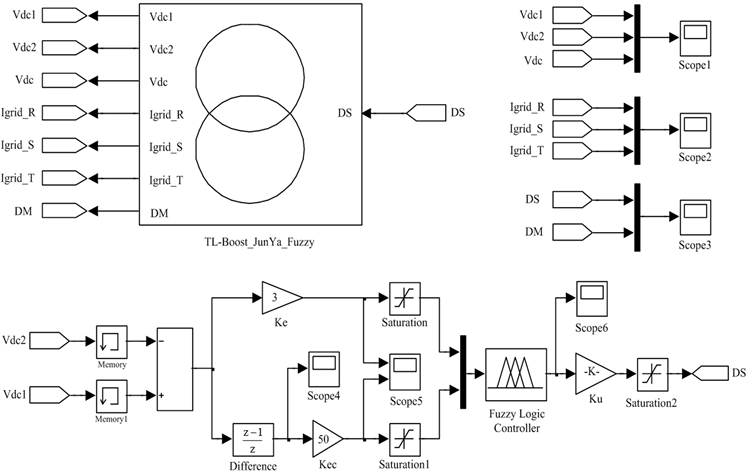(a) MATLAB/Simulink仿真模型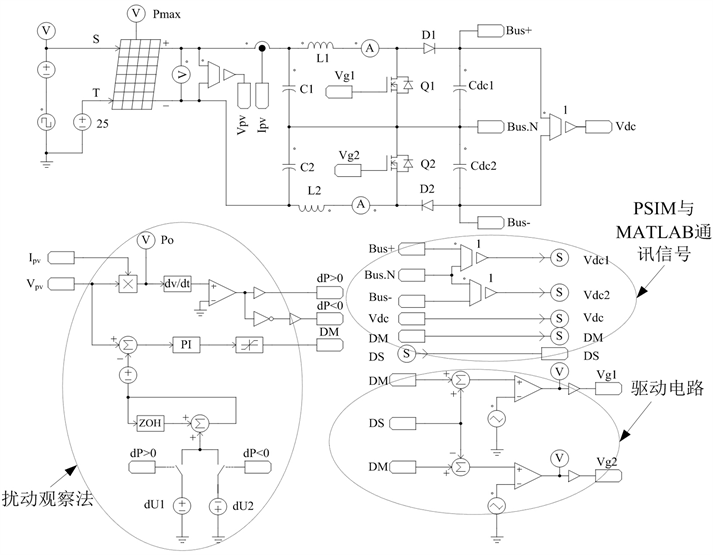(b) PSIM中Boost电路仿真模型

Figure 6. Simulation model of fuzzy control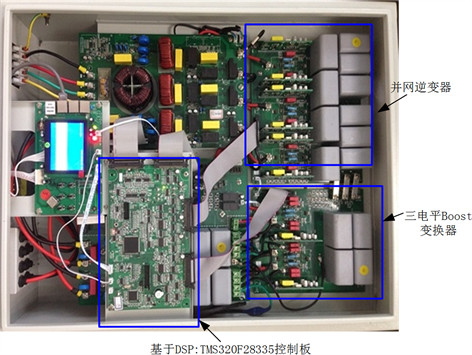Figure 7. 17 kW two-stage non-isolated three-phase grid-connected prototype based on three-level Boost converter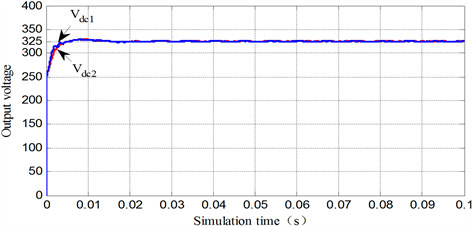Figure 8. Simulation waveforms with the same initial voltages across capacitors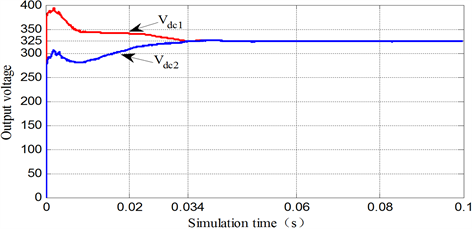Figure 9. Simulation waveforms with different initial voltages across capacitors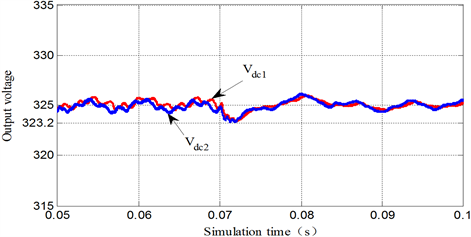Figure 10. Simulation waveforms when sudden increasing load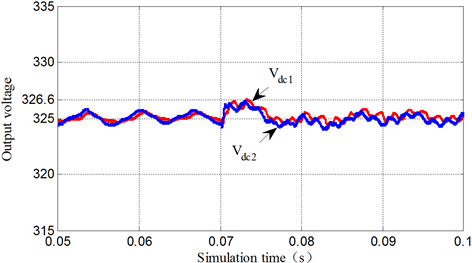Figure 11. Simulation waveforms when sudden decreasing load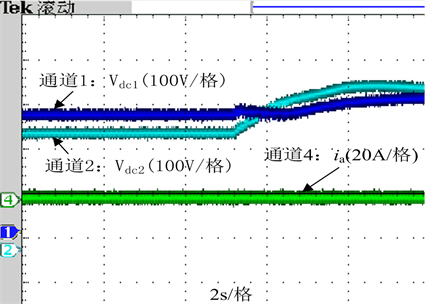Figure 12. Experimental waveforms of soft startwithout fuzzy controller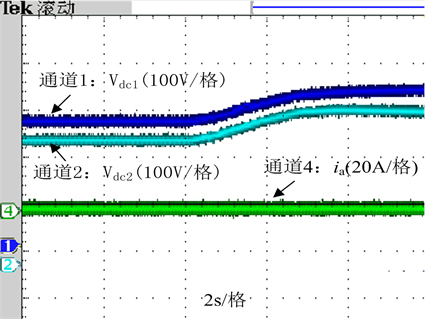Figure13. Experimental waveforms of soft startwith fuzzy controller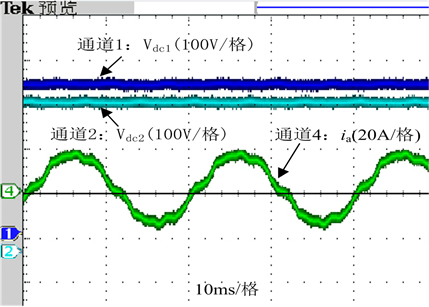Figure 14. Experimental waveforms in steady state with fuzzy controller

5. 结论

Output Capacitor Voltage Balance Control Method of Three-Level Boost DC-DC Converter Using Fuzzy Controller[J]. 电路与系统, 2019, 08(03): 56-66. https://doi.org/10.12677/OJCS.2019.8383008

1. 1. 臧壮, 潘朝峰, 陈宁, 邱广庭. 游艇燃料电池混合动力系统的模糊逻辑控制策略研究[J]. 船舶, 2017, 28(2): 65-70.

2. 2. 苗敬利, 郑大伟, 周重霞. 基于新型趋近律的永磁同步电机模糊滑模控制[J]. 电气传动, 2019, 49(3): 3-7.

3. 3. 郑玉强, 张航星, 任文, 涛张潮, 李涛. 基于Simulink的主动悬架模糊控制策略分析[J]. 机械工程与自动化, 2019(2): 89-91.

4. 4. 高夏翔, 张艳兵, 王水. 试件轴角位置跟踪控制技术的改进及仿真[J]. 电气传动, 2019, 49(3): 83-86.

5. 5. Yaramasu, V. and Wu, B. (2011) Three-Level Boost Converter Based Medium Voltage Megawatt PMSG Wind Energy Conversion Systems. 2011 IEEE Energy Conversion Congress and Exposition, Phoenix, 17-22 September 2011, 561-567. https://doi.org/10.1109/ECCE.2011.6063819

6. 6. Xia, C.L., Gu, X., Shi, T.N. and Yan, Y. (2010) Neutral-Point Potential Balancing of Three-Level Inverters in Direct-Driven Wind Energy Conversion System. IEEE Transactions on Energy Conversion, 26, 18-27. https://doi.org/10.1109/TEC.2010.2060487

7. 7. 张勤进, 刘彦呈, 王川. NPC光伏并网逆变器共模电流抑制方法研究[J]. 电机与控制, 2013, 17(8): 15-21.

8. 8. 童鸣庭, 苏建徽, 徐宏. 三电平中点电钳流位逆变器共模电压和电流抑制算法[J]. 低压电器, 2013(16): 32-37.